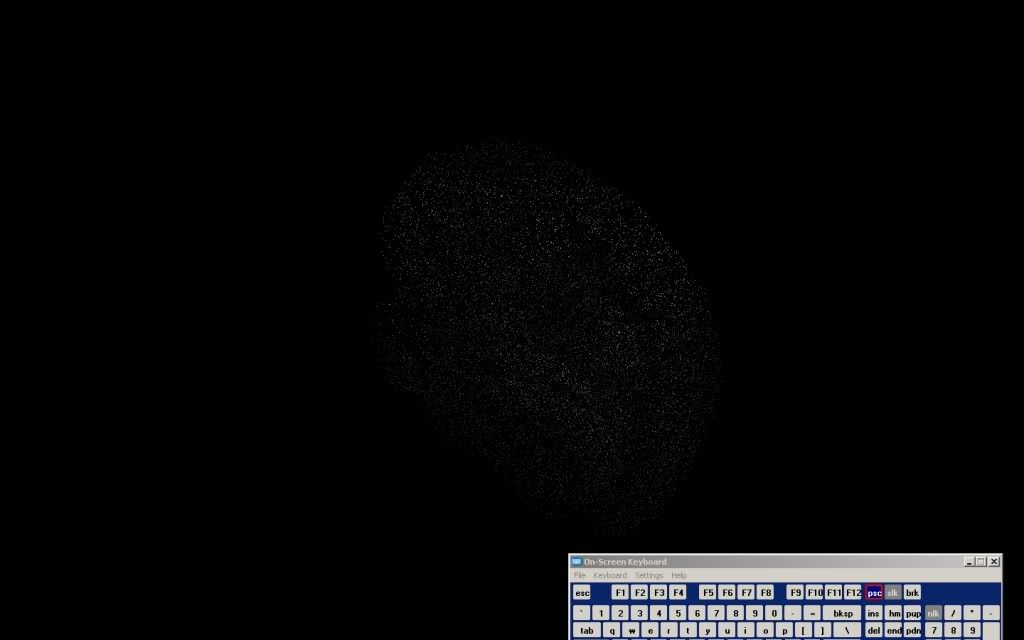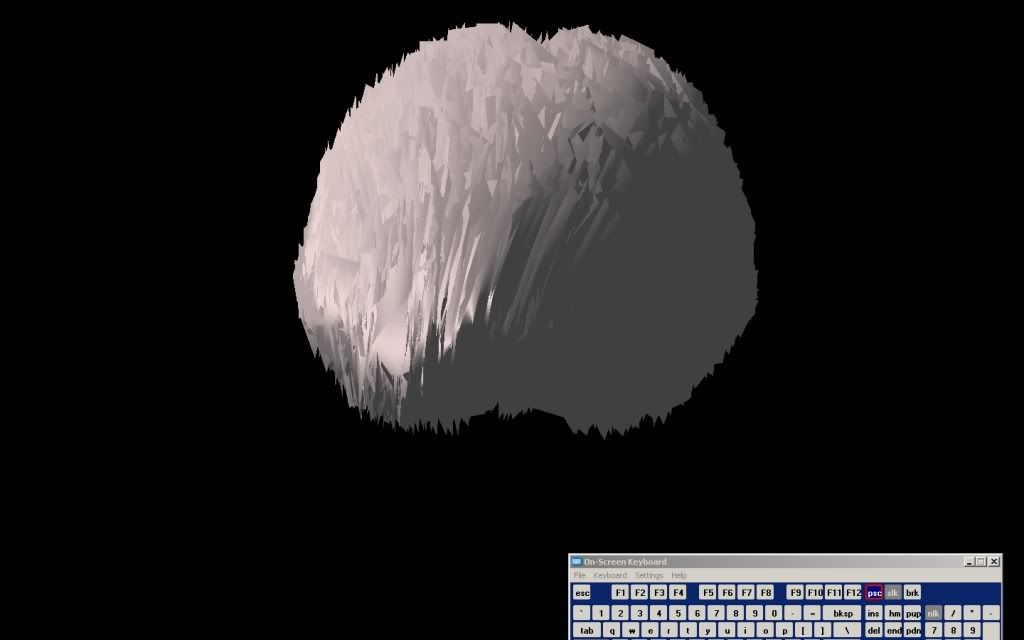# Vertex Buffer Object and Indices

I currently store all my vertices in an array[vertices3].
I then store a normal for each vertex in an array[vertices
3].
Finally, I have a given list of indexs cooresponding to the vertex. I am trying to use the index in a VBO in order to render.

My problem is, I believe, in the indices. Initially I am given a list of 10,000 vertices, each structure has an XYZ coordinate.
When I add this to the array[vertices*3], I insert array=vertex0.x, array=vertex0.y, array=vertex0.z, etc…

Now my initial indices don’t index directly to the vertices? An example is instead of the index 1 referencing the 2nd vertex it will reference the y coordinate of vertex0…

Why is this confusing me? Any help would be greatly appreciated!

When you setup your vertex array using glVertexPointer, you give a size parameter. GL uses this to know how many values to jump forward for each index.
So you’d give a size of 3 to glVertexPointer, and so index 0 would reference array, and index 1 will reference array.
Different attributes can have different sizes, and the index will still work correctly.

glVertexPointer(3, GL_FLOAT,3*sizeof(float), NULL);

That is called the stride, correct? If this is set correctly, when I view my model as only points, all the points show up correctly. The problem when connecting these is madness. Does this implicitly mean error in my indices? Or how I set up the indices in the c++ code?

Thanks for the quick response before!

Well its a combination of the stride and the size, depending on their values.
A stride of 0 is the same as a stride of (size * sizeof(thedatatype)). 0 is a special value which means the value are tightly packed (stride = size * sizeof(thedatatype)).

If you have some other stride (like if your data is in a single array, vx, vy, vz, nx, ny, nz), then it’ll jump forward ‘stride’ bytes every index.

It does sound like the issue is the connectivity, since the points are rendering correctly.

Can you look these two screenshots over:As you can see the points are where they should be! Luckily! But the problem arises from connecting them. Since I’m pretty new to openGL I could go for any suggestions on debugging this. I don’t know how the indices themselves could be wrong, MATLAB uses them to render points.

Solved

MATLab indices start at 1, not 0.

I hope this can help someone in the future from a terrible annoyance!

Nice!I was short of ideas…